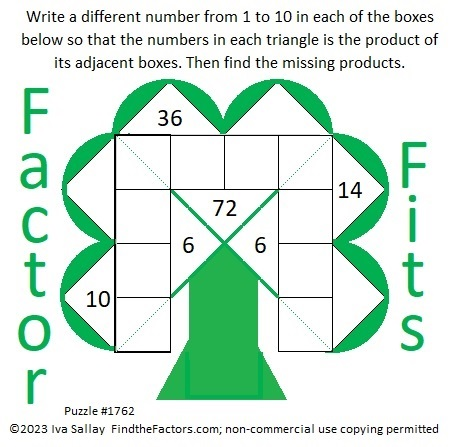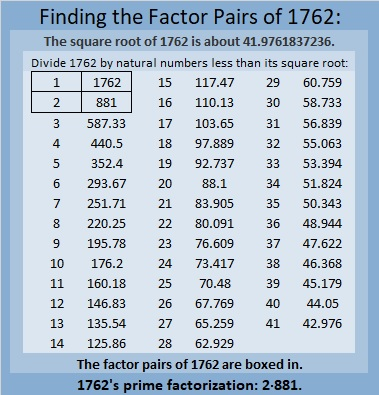# 1762 Happy Saint Patrick’s Day!

### Today’s ♣ Puzzle:

Here’s a much easier puzzle than yesterday’s for you to enjoy on this Saint Patrick’s Day. The diagonal lines on the corner boxes are only to help define the leaves of the shamrock.### Factors of 1762:

• 1762 is a composite number.
• Prime factorization: 1762 = 2 × 881.
• 1762 has no exponents greater than 1 in its prime factorization, so √1762 cannot be simplified.
• The exponents in the prime factorization are 1 and 1. Adding one to each exponent and multiplying we get (1 + 1)(1 + 1) = 2 × 2 = 4. Therefore 1762 has exactly 4 factors.
• The factors of 1762 are outlined with their factor pair partners in the graphic below.### More About the Number 1762:

1762 is the sum of two squares:
41² + 9² = 1762.

1762 is the hypotenuse of a Pythagorean triple:
738-1600-1762 calculated from 2(41)(9), 41² – 9², 41² + 9².
It is also 2 times (369-800-881).

1762 is palindrome 7C7 in base 15
because 7(15²) + 12(15) + 7(1) = 1762.